# HashSet in Java Example | Java HashSet Tutorial

Java HashSet is an inbuilt class that is used to create a collection that uses the hash table for storage. It inherits the AbstractSet class and implements the Set interface. Whenever it comes to storing data the primary concern is which data structure would be the most efficient to store and compute data and in this, we will discuss one of the most fundamental data structures in the Java Collections API efficient HashSet it is in terms of usability given its constant-time performance and ability to avoid duplicates.

## HashSet in Java Example

Java HashSet class implements the Set interface, backed by a hash table which is actually a HashMap instance. No guarantee is made as to the iteration order of the set which means that the class does not guarantee the constant order of elements over time. This class permits the null element.

The important points about the Java HashSet class are:

1. HashSet doesn’t maintain insertion order. Elements are inserted based on the hashcode.
2. HashSet is the best approach to search for the operations.
3. The initial default capacity of the HashSet is 16, and the load factor is 0.75.
4. HashSet stores the items by using the mechanism called hashing.
5. HashSet allows the null value.
6. The HashSet class is non synchronized.
7. HashSet contains unique elements only.

## #Constructors of Java HashSet class

### #HashSet()

It is used to construct the default HashSet.

### #HashSet(int capacity)

It is used to initialize a capacity of a hash set to the given integer value capacity. The capacity grows automatically as the elements are added to the HashSet.

It is used to initialize a capacity of the hash set to the given integer value capacity and the specified load factor.

### #HashSet(Collection<? extends E> c)

It is used to initialize a hash set by using the items of the collection c.

## #Example of HashSet in Java

```import java.util.ArrayList;
import java.util.HashSet;
import java.util.Iterator;
import java.util.List;
import java.util.Set;

class HashSetExample {
public static void main(String[] args) {
HashSet<String> Heroes = new HashSet<>();// creating hashset

System.out.println(Heroes);

Heroes.remove("Batman");

Iterator<String> i = Heroes.iterator();
while (i.hasNext()) {
System.out.println(i.next());
}

}
}```

See the following output.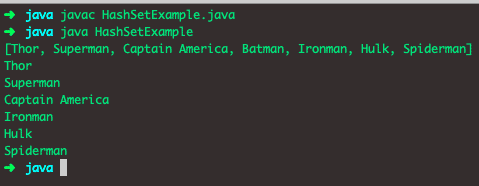## #Major Aspects of its Implementation

1. Hash-Set cannot contain duplicate keys which enables it to handle only data values that are unique and allow NULL values.
2. It’s not thread-safe as Multi-threading is one of Java’s main features; basically, you have multiple threads, accessing the same data, from a single object. This often can lead to inconsistency when the threads read and update the shared data.
3. Insertion order is not maintained in the HashSet, and It is an unordered collection. It Implements the SET Interface.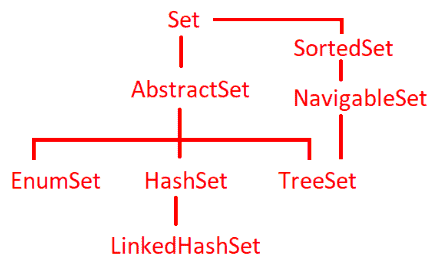## #HashSet Hierarchy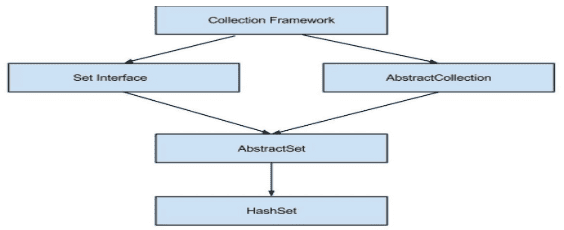## #Creation of HashSet and adding elements

The creation of the hash set and adding elements in it is shown through this example in java where elements are added in it via the add() method.

```import java.util.ArrayList;
import java.util.HashSet;
import java.util.Iterator;
import java.util.List;
import java.util.Set;

public class HashSetExample {

public static void main(String[] args) {

Set<String> Program_Languages = new HashSet<>();

System.out.println(Program_Languages);
}
}```

### #Output of Program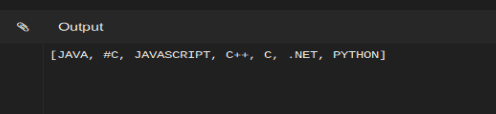## #Methods on HashSet

The add() function adds the given item to the Set if not already present. This method internally uses the equals() method to check for duplicates.

### #clear():

Removes all the elements from the Set.

### #clone():

Returns the shallow copy of the Set instance.

### #contains(Object o):

Returns true if the Set contains the given element, otherwise false.

See the following code.

```import java.util.ArrayList;
import java.util.HashSet;
import java.util.Iterator;
import java.util.List;
import java.util.Set;

public class HashSetExample {

public static void main(String[] args) {

Set<String> Program_Languages = new HashSet<>();

// contains example
System.out.println(" Program_Languages contains C= " + Program_Languages.contains("C"));
System.out.println(" Program_Languages contains RUBY = " + Program_Languages.contains("RUBY"));

}
}```

See the following output.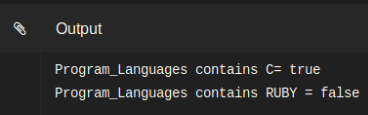### #isEmpty():

Returns true if Set contains no elements, otherwise false.

See the following code.

```import java.util.ArrayList;
import java.util.HashSet;
import java.util.Iterator;
import java.util.List;
import java.util.Set;

public class HashSetExample {

public static void main(String[] args) {

Set<String> Program_Languages = new HashSet<>();

System.out.println("Program_Languages set is empty = " + Program_Languages.isEmpty());
}
}```

See the following output.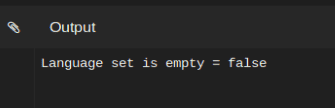### #iterator()

Returns the iterator over the items in this set. The items are returned in no particular order. See the following code example.

```import java.util.ArrayList;
import java.util.HashSet;
import java.util.Iterator;
import java.util.List;
import java.util.Set;

public class HashSetExample {

public static void main(String[] args) {

Set<String> Program_Languages = new HashSet<>();

// iterator example
Iterator<String> iterator = Program_Languages.iterator();
while (iterator.hasNext()) {
System.out.println("Learning Program_Languages " + iterator.next());
}

}

}```

See the following output.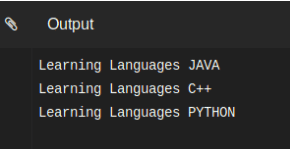### #remove(Object o)

Removes the given item from this set if it is present and returns true. If the item is not present in the set, returns false.

See the following code.

```import java.util.ArrayList;
import java.util.HashSet;
import java.util.Iterator;
import java.util.List;
import java.util.Set;

public class HashSetExample {

public static void main(String[] args) {

Set<String> Program_Languages = new HashSet<>();

// remove
System.out.println("PYTHON removed from fruits set = " + Program_Languages.remove("PYTHON"));
System.out.println("C removed from fruits set = " + Program_Languages.remove("C"));
System.out.println(Program_Languages);
}
}```

See the following output.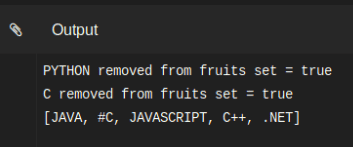### #size()

Returns a number of elements in the set.

```import java.util.ArrayList;
import java.util.HashSet;
import java.util.Iterator;
import java.util.List;
import java.util.Set;

public class HashSetExample {

public static void main(String[] args) {

Set<String> Program_Languages = new HashSet<>();

// size example
System.out.println(" Program_Languages set size = " + Program_Languages.size());
}
}```

See the following output.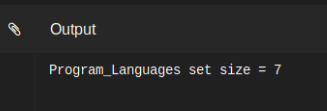### #spliterator()

Creates the late-binding and fail-fast Spliterator over the items in this set. This is introduced in Java 8.0

### #removeAll(Collection<> c)

Hash-Set inherits this method from Abstract-Set. This method will remove all the elements in the set that are part of the specified collection.

## #Creating a Hash-Set from another collection

We can create a hash set from another collection as a list, and through the “addAll” method, we can form a hash set. See in the given example.

```import java.util.ArrayList;
import java.util.HashSet;
import java.util.Iterator;
import java.util.List;
import java.util.Set;

public class HashSetExample {

public static void main(String[] args) {

Set<String> Program_Languages = new HashSet<>();

List<String> list = new ArrayList<>();

System.out.println("Program_Languages set before addAll = " + Program_Languages);
System.out.println("list = " + list);
System.out.println("Program_Languages set after addAll = " + Program_Languages);
}
}```

See the following example.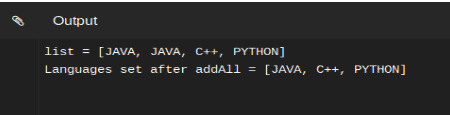We can observe that addall method does not allow to insert duplicate in the set.

## #Performance on HashSet

One of the important things of importance, when one is using a data structure, is efficiency by which operations are possible in it.

The expected time complexity of inserting an item to a set is O(1), which can drop to O(n) in the worst-case scenario.

IMP NOTE: since JDK 8, the worst-case time complexity is O(log*n).

The performance of a Hash-Set is affected mainly by two parameters – its Initial Capacity and the Load Factor.

## #Comparison of LIST and SET in terms of Runtime

List and HashSet are often compared, and search performance of the generic HashSet<T> class is higher than of the generic List<T> class. First, compare the hash-based key and another one with the linear approach in the List<T> class, but an important point is if your collection is so small than a List is faster.### #How HashSet maintain uniqueness(Internal working)

HashSet utilizes Hash-Map for storing its elements. HashSet works with equals() and hashCode() method to check for the duplicate element when you try to add an item. See the following code.

```import java.util.ArrayList;
import java.util.HashSet;
import java.util.Iterator;
import java.util.List;
import java.util.Set;

public class HashSetExample {

public static void main(String[] args) {

Set<Emp> emps = new HashSet<>();
System.out.println(emps);
}

}
class Emp {
private String Language;
private int id;

public Emp(int i, String n) {
this.id = i;
this.Language = n;
}

@Override
public String toString(){
return "{"+id+","+Language+"}";
}
}```

In the above example code we are not implementing equals()method in the set so duplicate keys are inserted.

## #How HashSet maintain uniqueness

HashSet utilizes HashMap for storing its elements. HashSet works with equals() and hash method to check for the duplicate element when you try to add an element. See the following code program.

```import java.util.ArrayList;
import java.util.HashSet;
import java.util.Iterator;
import java.util.List;
import java.util.Set;

public class HashSetExample {

public static void main(String[] args) {

Set<Emp> emps = new HashSet<>();

System.out.println(emps);
}

}
class Emp {
private String Language;
private int id;

public Emp(int i, String n) {
this.id = i;
this.Language = n;
}

@Override
public String toString(){
return "{"+id+","+Language+"}";
}
}```

In the below example code we are not implementing equals() method in the set so duplicate keys are inserted.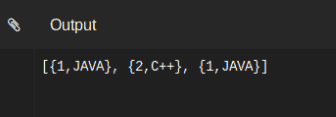This will give output with java added two times in the list, it’s happening because the Emp class doesn’t define equals() method, which does not allow duplicate values in the set.

Finally, HashSet in Java Example Tutorial is over.

This site uses Akismet to reduce spam. Learn how your comment data is processed.